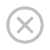# NumPy 简介

## 什么是 NumPy？

NumPy 是用于处理数组的 python 库。

NumPy 由 Travis Oliphant 于 2005 年创建。它是一个开源项目，您可以自由使用它。

NumPy 指的是数值 Python（Numerical Python）。

## 为何使用 NumPy？

NumPy 旨在提供一个比传统 Python 列表快 50 倍的数组对象。

NumPy 中的数组对象称为 ndarray，它提供了许多支持函数，使得利用 ndarray 非常容易。

## NumPy 用哪种语言编写？

NumPy 是一个 Python 库，部分用 Python 编写，但是大多数需要快速计算的部分都是用 C 或 C ++ 编写的。

## NumPy 代码库在哪里？

NumPy 的源代码位于这个 github 资料库中：https://github.com/numpy/numpy

github：使许多人可以在同一代码库上工作。